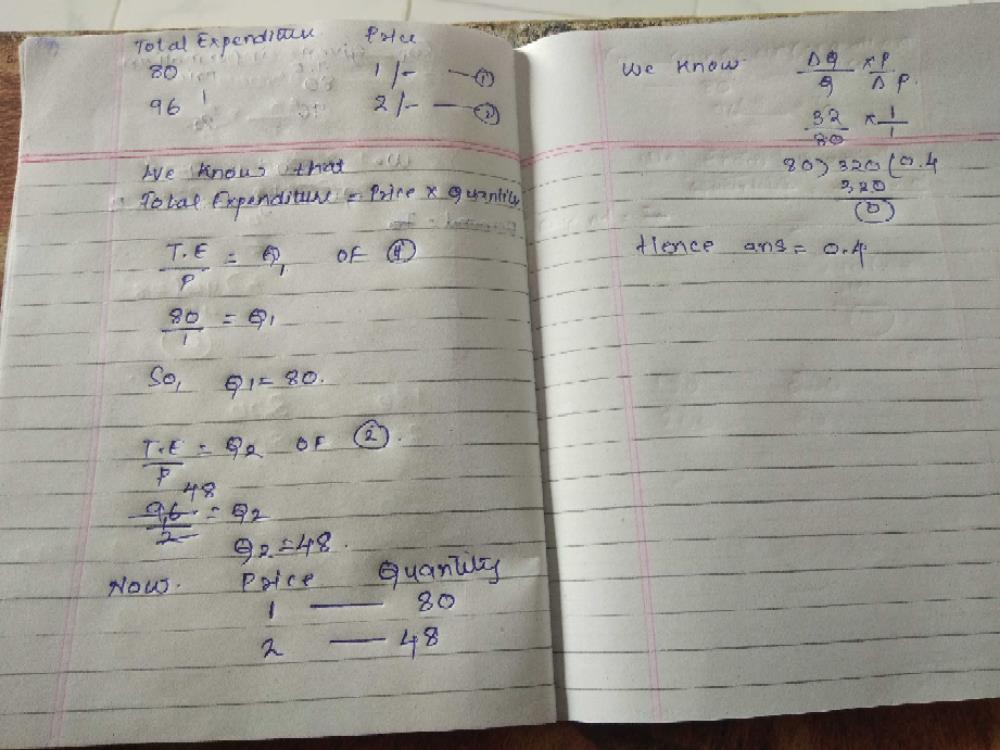Courses

# A consumer spends Rs. 80 on purchasing a commodity when its price is Re. 1 per unit and spends Rs. 96 when the price is Rs. 2 per unit. Calculate the price elasticity of demand. a)0.2b)0.3c)0.4d)0.5Correct answer is option 'C'. Can you explain this answer? Related Test: Test: Theory Of Demand- 3

## CA Foundation Question

By Dhanush Surya · Sep 24, 2018 ·CA Foundation
Chandna Arora answered Jun 05, 2018
Initial Total Expenditure (TEo)=Rs 80
Final Total Expenditure (TE1)=Rs 96
Initial Price (Po)=Rs 1
Final Price (P1)=Rs 2

Now, Quantity Q = TE/P
Qo = 80/1 = 80
Q1 = 96/2 = 48

Now,

Ed=(−)[Po/Qo] x [ΔQ/ΔP]

Ed=(−)1/80 x [48−80]/(2−1)
Ed=(−)1/80 x (−32/1)
Ed=(−)−0.4
Ed=0.4

Thus, the price elasticity of demand is 0.4.

Goplar Ajay answered Dec 23, 2018Shaheen Fatma answered Sep 24, 2018
80/96×2 =0.4

Dhanush Surya answered Jun 05, 2018
Any body explain this and how